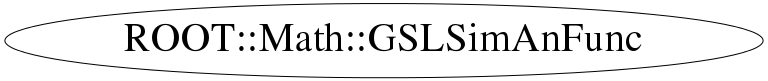# class ROOT::Math::GSLSimAnFunc

```
GSLSimAnFunc class description.
Interface class for the  objetive function to be used in simulated annealing
If user wants to re-implement some of the methods (like the one defining the metric) which are used by the
the simulated annealing algorithm must build a user derived class.
NOTE: Derived classes must re-implement the assignment and copy constructor to call them of the parent class

@ingroup MultiMin

```

## Function Members (Methods)

public:
 virtual ~GSLSimAnFunc() virtual ROOT::Math::GSLSimAnFunc* Clone() const virtual double Distance(const ROOT::Math::GSLSimAnFunc& func) const virtual double Energy() const virtual ROOT::Math::GSLSimAnFunc& FastCopy(const ROOT::Math::GSLSimAnFunc& f) ROOT::Math::GSLSimAnFunc GSLSimAnFunc(const ROOT::Math::GSLSimAnFunc&) ROOT::Math::GSLSimAnFunc GSLSimAnFunc(const ROOT::Math::IMultiGenFunction& func, const double* x) ROOT::Math::GSLSimAnFunc GSLSimAnFunc(const ROOT::Math::IMultiGenFunction& func, const double* x, const double* scale) unsigned int NDim() const ROOT::Math::GSLSimAnFunc& operator=(const ROOT::Math::GSLSimAnFunc&) virtual void Print() double Scale(unsigned int i) const void SetX(const double* x) void SetX(unsigned int i, double x) virtual void Step(const ROOT::Math::GSLRandomEngine& r, double maxstep) const vector& X() const double X(unsigned int i) const
protected:

## Data Members

private:
 const ROOT::Math::IMultiGenFunction* fFunc vector fScale vector fX

## Class Charts## Function documentation

GSLSimAnFunc(const ROOT::Math::IMultiGenFunction& func, const double* x)
```      construct from an interface of a multi-dimensional function

```
GSLSimAnFunc(const ROOT::Math::IMultiGenFunction& func, const double* x, const double* scale)
```      construct from an interface of a multi-dimensional function
Use optionally a scale factor (for each coordinate) which can  be used to scale the step sizes
(this is used for example by the minimization algorithm)

```

```      derived classes might need to re-define completely the class

```
`{}`
virtual ~GSLSimAnFunc()
``` virtual distructor (no operations)
```
`{ }`
GSLSimAnFunc & FastCopy(const ROOT::Math::GSLSimAnFunc& f)
```      fast copy method called by GSL simuated annealing internally
copy only the things which have been changed
must be re-implemented by derived classes if needed

```
GSLSimAnFunc * Clone() const
```      clone method. Needs to be re-implemented by the derived classes for deep  copying

```
return new GSLSimAnFunc(const ROOT::Math::GSLSimAnFunc& )
double Energy() const
```      evaluate the energy ( objective function value)
re-implement by derived classes if needed to be modified

```
void Step(const ROOT::Math::GSLRandomEngine& r, double maxstep)
```      change the x[i] value using a random value urndm generated between [0,1]
up to a maximum value maxstep
re-implement by derived classes if needed to be modified

```
double Distance(const ROOT::Math::GSLSimAnFunc& func) const
```      calculate the distance (metric) between  this one and another configuration
Presently a cartesian metric is used.
re-implement by derived classes if needed to be modified

```
void Print()
```      print the position in the standard output std::ostream
GSL prints in addition n iteration, n function calls, temperature and energy
re-implement by derived classes if necessary

```
void SetX(const double* x)
```       change the x values (used by sim annealing to take a step)

```
void SetX(unsigned int i, double x)
unsigned int NDim() const
`{ return fX.size(); }`
double X(unsigned int i) const
`{ return fX[i]; }`
const std::vector<double> & X() const
`{ return fX; }`
double Scale(unsigned int i) const
`{ return fScale[i]; }`